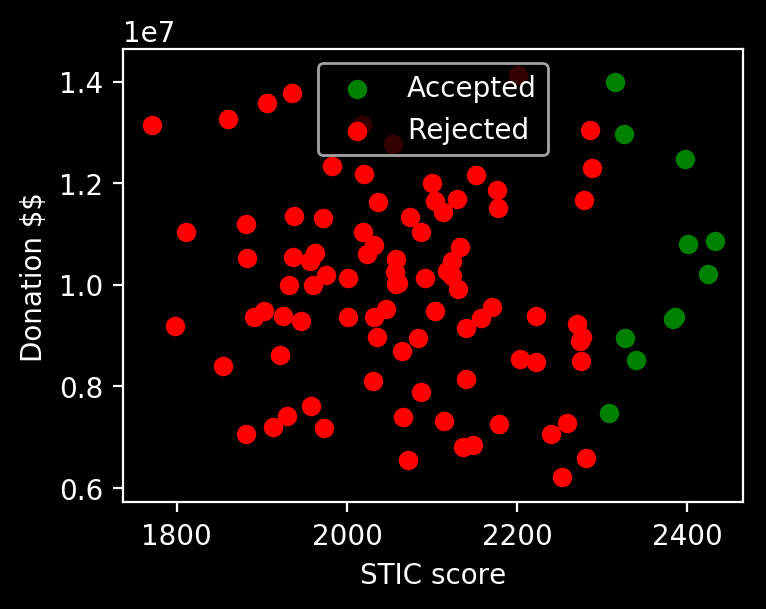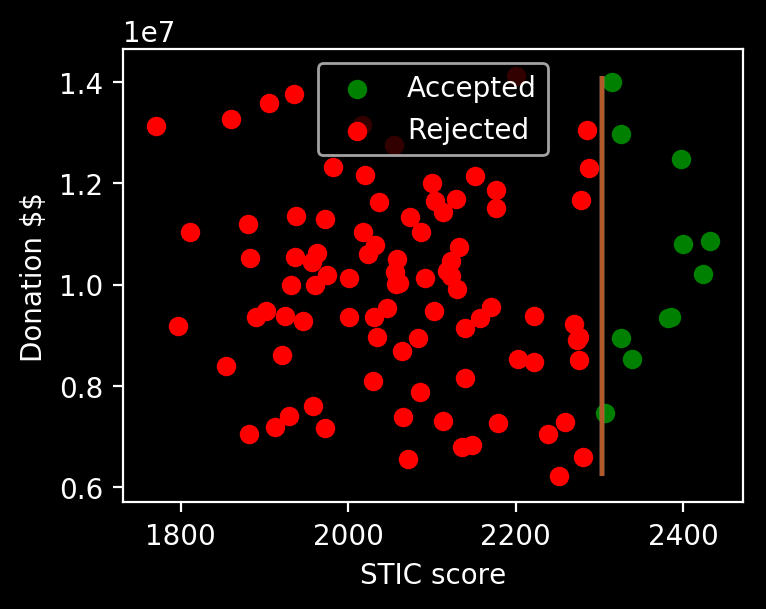# Stats and Bonuses

Jim's blog on data and everything else | 🔮💰👑🤔💻📷🍁🌌🔬🏹

# Machine Learning: Basics

## Typical scenario for Machine Learning¶

• There exists some phenomenon called an unknown target function in the world which produces a measurement 'label' from some observations 'features'. We don't know this target function, and probably never will, but we have data.
• Note: produce need not imply any causal relationships. Feature: 'it is raining' can produce a label: 'there are dark clouds', even though dark clouds cause rain
• We have some examples of [feature, label] pairs and want to learn an approximated target function, which represents our understanding of the world
• To do this, our machine trains on the examples and modifies its approximation until we are satisfied
• To assess the machine, we give it test data it has never seen before and force it to run the approximated target function on the features, producing predicted labels. If the predicted labels are the same as the true labels, then we have a good approximation

### Human Learning analogy¶

• You go to school and your teacher shows you a textbook problem ('features')
• Initially, you can't do them (untrained model)
• You go through 50 of the same type of problem (training the model)
• You sit your final exam and you are given a grade (testing the model)

## Example of supervised learning¶

First, we need some fake data and a scenario. I will use Python. I like using Anaconda's Spyder for Python, since it feels like RStudio and Matlab.

### The fake scenario will be generated as follows:¶

• We have a world-class university called Mass Tech Haabad that accepts students under the condition 'STIC score must be greater than 2300'. Only they know this fact. This is the target function.
• Haabad doesn't care about donations, race, gender, sexual orientation, athletics, etc.
• I call this bullshit fake data because such a university does not exist
• This is a severe issue that plagues liberal US universities, and schools like Cambridge are much closer to the ideal
• As a high schooler, we will collect perfect data of 100 past applicants from College Confidential. We will look at [STIC score, money donated] ($X$, or 'features'), and whether they got in or not ($y$, or 'labels'). Data will be stored as a 2-dimensional array with 100 entries: [[STIC1, donation1], [STIC2, donation2], ...]
• We want to try and learn the target function by which Haabad selects applicants.

### College Confidential data is sampled from Gaussian distributions with mean=2100, std=200 for STIC scores and mean=10,000,000, std=2,000,000 for donations.¶

In :
import numpy as np # numpy does numbers
import pandas as pd # super convenient data handler
stic = np.random.normal(loc=2100,
scale=200,
size=100)
money = np.random.normal(loc=1e7,
scale=2e6,
size=100)
# concatenate the two observations
X = np.stack([stic, money], axis=1)
# X now looks like:
# [[STIC1, donation1]
#  [STIC2, donation2]
#  [STIC3, donation3]
#  ...
#  [STIC100, donation100]]
df = pd.DataFrame(X) # turn into dataframe
df.columns = ['stic', 'money']

# calculate: did they get in? True or False?
# so just test whether each STIC is > 2300
df['result'] = df.stic>2300


          stic         money  result
0  2177.243163  1.151015e+07   False
1  1981.832327  1.232999e+07   False
2  1882.705049  1.052641e+07   False
3  2060.365527  1.002570e+07   False
4  2071.366867  6.552017e+06   False


### Now we plot the X and y on a scatter plot and see the results.¶

In :
import matplotlib.pyplot as plt # plotting package
import matplotlib as mpl
mpl.style.use('dark_background')

plt.scatter(df[df.result==True]['stic'],
df[df.result==True]['money'],
color='green', label='Accepted')
plt.scatter(df[df.result==False]['stic'],
df[df.result==False]['money'],
color='red', label='Rejected')
plt.xlabel('STIC score')
plt.ylabel('Donation \$\$')
plt.legend()
fig = plt.gcf()
fig.set_dpi(200)
fig.set_size_inches(4,3, forward=True)
plt.show()
plt.close()We observe that we can probably separate rejections and acceptances with a straight line. So, we train a neural network line separator (linear support vector classifier) on the data we have plotted.

Importantly, we want to assess the line that we learn, so we will make a 1000x1000 meshgrid of [STIC, donation] points and see where the decision boundary is. Since we know the true acceptance rule for Haabad (target function: STIC>2300?), we can just eyeball the line that we draw.

In :
from sklearn import svm
from sklearn.preprocessing import StandardScaler
# need to standardise data
# define our linear model and training parameters
SS = StandardScaler()
model = svm.LinearSVC(C=1e1)
# features are X
X = SS.fit_transform(df[['stic', 'money']])
# labels are y
y = df['result']*1
# train our model
model.fit(X, y)

# see what our line is like
# make a meshgrid and evaluate SVM in there
h = 1000  # number of meshpoints each dimension
x_min, x_max = X[:, 0].min(), X[:, 0].max()
y_min, y_max = X[:, 1].min(), X[:, 1].max()
xx, yy = np.meshgrid(np.linspace(x_min, x_max, h),
np.linspace(y_min, y_max, h))
Z = model.predict(np.c_[xx.ravel(), yy.ravel()])

# actually want to plot unstandardised
X = df[['stic', 'money']].values
x_min, x_max = X[:, 0].min(), X[:, 0].max()
y_min, y_max = X[:, 1].min(), X[:, 1].max()
xx, yy = np.meshgrid(np.linspace(x_min, x_max, h),
np.linspace(y_min, y_max, h))

# Put the result into a color plot
Z = Z.reshape(xx.shape)

plt.contour(xx, yy, Z, cmap=plt.cm.Paired)
plt.scatter(df[df.result==True]['stic'],
df[df.result==True]['money'],
color='green', label='Accepted')
plt.scatter(df[df.result==False]['stic'],
df[df.result==False]['money'],
color='red', label='Rejected')
plt.xlabel('STIC score')
plt.ylabel('Donation \$\$')
plt.legend()
fig = plt.gcf()
fig.set_dpi(200)
fig.set_size_inches(4,3, forward=True)
plt.show()
plt.close()Our support vector linear classifier correctly classifies the training points perfectly and also appears to learn the true target function boundary at STIC=2300, according to the 1000x1000 meshgrid of [STIC, donation] pairs (test data). We want to see whether we fell into any traps:

### Typical learning pitfalls¶

1. Test data comes from a different distribution as the training data: you studied for Physics, but your exam is on Finance
2. Overfitting on the training data with a powerful model: you memorise the textbook answers but don't know how to do them. You see a similar problem with different numbers on the exam, but don't know what to do
3. Training on the test data: you stole the final exam, and the solutions too
4. Testing on training data: your final exam is the textbook, of course you do well
5. Training on (unavailable) future data: when your job is to predict tomorrow's stock prices or the rain, you accidentally look into a crystal ball during training
6. Test data snooping: you have a good idea of what the test data looks like already, so you pick a model that can generate the test data

The only thing we care about is out-of-sample (quiz/test) performance. Training performance is not testing performance. No matter how well you learned the textbook material, the final exam is the only thing that matters to companies your self-worth. Out-of-sample performance is the name of the game

### We're kind of ok, for now¶

1. Our test data is the meshgrid of points. It's not the same distribution for sure, but we perform just fine
2. Our model (a line) isn't very powerful, so it can't memorise things easily
3. We didn't make the meshgrid until after training (model.fit(X, y))
4. The training points are a small fraction of the size of the 1000x1000 meshgrid, so it's ok
5. All of our data was collected before we trained
6. We not only snooped on the test data, we generated the test data. We wrote and took our own final exam. But that is the problem with fake data, and there is no getting around it

## Realistic example for ML¶

• Suppose there are 12,000 ID photos from the local police department of a very homogeneous population (ages 18-30, no beard, all male, same race, same photographer, etc.)
• For 10,000 of them, police dept. tells you which ones are convicted criminals (50% of them are)
• The other 2,000 don't have labels, but the dept. will reveal them in a year
• Target function: we don't know if it exists in reality, but we want to take an ID photo and return True or False for that person being a convicted criminal
• Features: just the ID photos. Label: convicted criminal? True or False
• If we have code that gets more than 80% accuracy on the 2,000 unlabeled pictures, we will have made a groundbreaking discovery for sure

## Can we use machine learning? 3 questions:¶

1. Is there anything to learn? We can't learn a coin toss
2. Do we have an exact formula of the target function that we can evaluate? If so, then we just use that formula
3. Do we have data?

## So what happens when we have more complex target functions and how do we quantify whether a model is 'good', aside from staring at it?¶

Overfitting and Cross Validation on the next post :)